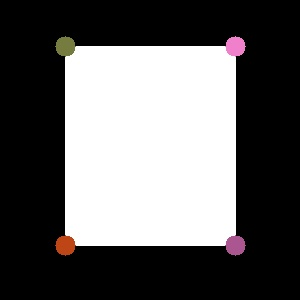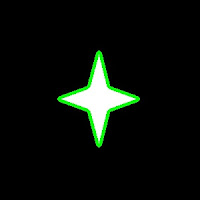OpenCV-Python

OpenCV Python Tutorials

opencvpython.blogspot.com

Contours - 1 : Getting Started

Hi, this article is a tutorial which try to cover all relevant functions in OpenCV dealing with Structural Analysis and Shape Descriptors, which are mainly related to contours.Contours can be explained simply as a curve joining all the continuous points (along the boundary), having same color or intensity. For example, consider image at left.

Assuming it is a binary image,we can say, its contour is the curve joining the all the boundary white points.

So if we find a contour in a binary image, we are finding the boundaries of objects in an image. That is why, OpenCV doc says, "The contours are a useful tool for shape analysis and object detection and recognition".

Finding Contours:

We start with a simple image as above. First we find the contours.

import numpy as np
import cv2

imgray = cv2.cvtColor(im,cv2.COLOR_BGR2GRAY)
ret,thresh = cv2.threshold(imgray,127,255,0)
contours, hierarchy = cv2.findContours(thresh,cv2.RETR_TREE,cv2.CHAIN_APPROX_SIMPLE)

Points to remember :
1. For better accuracy, use binary images. So before finding contours, apply threshold or canny edge detection.
2. FindContours function modifies the source image, so 'thresh' before and after finding contours are different image. So if you want 'thresh' as such after finding contours, store it to some other variables.
3. In OpenCV, its operation is like finding white object from black background. So remember, object to be found should be white and background in black.
What is structure of resulting contours?

The result "contours" is a Python list, where it contains all objects boundary points as separate lists. So to find number of objects, find length of list "contours", where in this case, it is one. Only one object. So we take it as "cnt".

>>> len(contours)
1
>>> cnt = contours
>>> len(cnt)
244

Here, number of points in cnt is 244. What these points denote? They are the boundary points of the object.

But, does it include all the boundary? Not exactly. The points are selected such that, contours can be drawn as straight line joining these points. So, if object is a horizontal or vertical line, only end points are stored. ie length of cnt = 2. If object is a rectangle, only 4 vertices are stored.Contour points for a rectangle

Thus in our image, there are no direct horizontal or vertical lines. So most of the points will be stored. To visualise it as above, you can draw circles for each value in cnt.

How to draw the contours?

For this, there is a function, cv2.drawContours(). Let's try it:

cv2.drawContours(im,contours,-1,(0,255,0),3)

This draws a 3-pixel wide green outline of the object. If you want to fill the object with a particular color, pass value of -1 to line thickness.

cv2.drawContours(im,contours,-1,(0,255,0),-1)Contours drawn filledContours drawn 3 px wide

Also, the third argument in cv2.drawContours() is also to be noted. Suppose, you want to draw only fourth contour(not here), third argument should be set to 3. If it is -1, all contours are drawn.

Now you want to draw "cnt" only. It can be done as follows:

cv2.drawContours(im,[cnt],0,(255,0,0),-1)

Note the square bracket around "cnt". Third argument set to 0, means only that particular contour is drawn.

Now we end after one more important concept, called Mask.

Mask can be considered as a binary image where only our desired area is white and all others are blacked out. They are used to isolate a part of image and do operations on that part only without affecting or operating on other parts of the image. This can also be considered as a ROI (Region of Interest) which can have any shape.Consider a scenario, where you are asked to find average colors of each shapes in the image at right. So simply threshold the image to binarize it (please don't ask me if white ball can be detected using thresholding, it is just an example). Find contours in the binary image, then for each contour, create a mask image of that shape. ie, if first ball is cosidered, the region of that ball in mask image will be white, while all other shapes and backgrounds are blacked out. Now if you can find the mean color of that shape only. So for every shapes.

(OK, just for this case, I will do it in this image, not on our original image at the beginning)

First we find the contours as we did before. (Adjust the threshold value to detect all). Now we will see how to do it:

First create a mask image where all elements are zero (ie just a black image) with size same as source, but single channel (ie grayscale).

Then for each contour, we draw it on the mask image filled with white color. Then we find mean using mean() function, taking our mask as operating mask.

for h,cnt in enumerate(contours):Mask Images

See the result at left side.

(All the resulting images are animated to a single image)

I think it is sufficient for now. Keep these three in mind, ie Find Contours, Draw Contours and Mask Image. Now we can find some contour features in next post.

Regards,
ARK

Install OpenCV in Windows for Python

Hi Friends,

Here I will tell you how to install OpenCV 2.4x in Windows  for Python 2.7.

Install:

1)  First install Python 2.7. Leave all settings as default. In that case, Python will be installed in default folder C:\Python27\

2) Now install Numpy. Again leave everything default. Numpy will find Python directory and will be installed to most appropriate folder.

3) Now double-click OpenCV.exe. It will ask for extraction folder. Give it as just C:\. It will extract all files to C:\opencv\  . Wait until everything is extracted.

4) Now copy everything in the folder C:\opencv\build\python\x86\2.7\ ( most probably, there will be only one file cv2.pyd ) and paste it in the folder C:\Python27\Lib\site-packages\

5) Now open your "Python IDLE" ( from Start > All Programmes > Python 2.7 > Python IDLE ) and just type following :
import cv2

If everything OK, it will import cv2 module, otherwise an error message will be shown.

So it is very simple procedure. Try it yourself and let me know how it goes.

NB : Even if you are using 64-bit windows, do the same procedure. ( Better don't go for 64-bit Python and Numpy )

With Regards,
ARK

Report "OpenCV-Python"

Are you sure you want to report this post for ?

Cancel
×